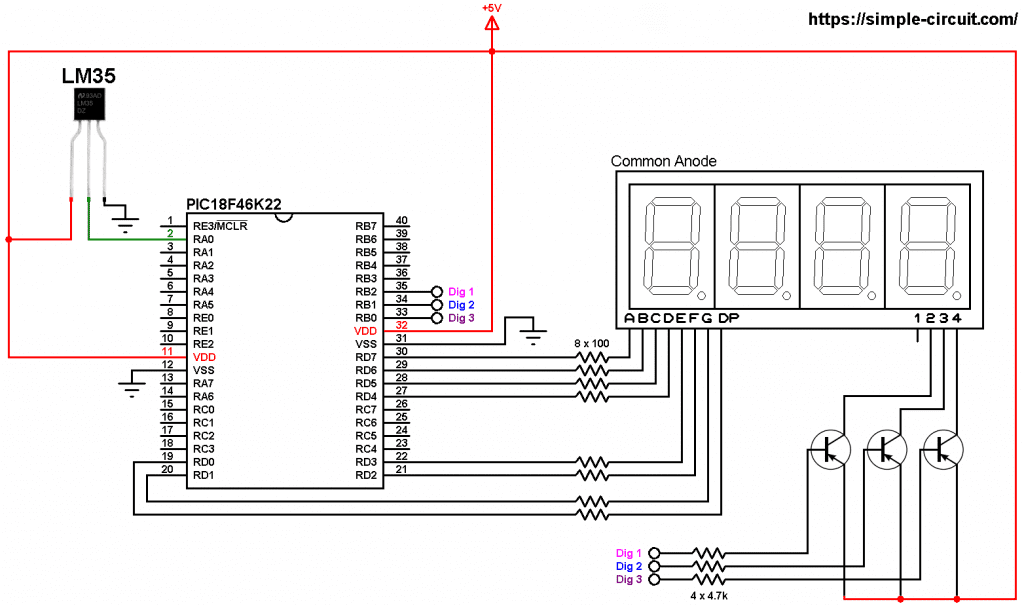# PIC18F46K22 with LM35 sensor and 7-segment display | mikroC Projects

This post shows how to make a temperature measurement station using PIC18F46K22 microcontroller and LM35 analog temperature sensor where temperature value is displayed on a three-digit common anode seven-segment display.
The compiler used in this example is mikroC PRO for PIC.

The LM35 temperature sensor is a three pin device (VCC, OUT and GND) with an output voltage linearly related to Centigrade temperature. Since the LM35 output varies with dependent to the temperature, we need an ADC (Analog-to-Digital Converter) module to measure this voltage. The PIC18F46K22 microcontroller has one ADC module with 10-bit resolution.

The LM35 output has linear +10mV/°C scale factor means the following:
If the output voltage =   10mV —> temperature =   1°C
If the output voltage = 100mV —> temperature = 10°C
If the output voltage = 200mV —> temperature = 20°C
If the output voltage = 370mV —> temperature = 37°C
and so on.

LM35 Futures (from datasheet):

• Calibrated Directly in ° Celsius (Centigrade)
• Linear + 10 mV/°C Scale Factor
• 0.5°C Ensured Accuracy (at +25°C)
• Rated for Full −55°C to +150°C Range
• Suitable for Remote Applications
• Low Cost Due to Wafer-Level Trimming
• Operates from 4 to 30 V
• Less than 60-μA Current Drain
• Low Self-Heating, 0.08°C in Still Air
• Nonlinearity Only ±¼°C Typical
• Low Impedance Output, 0.1 Ω for 1 mA Load

The ADC module converts analog data into digital data. The PIC18F46K22 MCU has a 10-bit ADC module and a built-in fixed voltage reference (FVR) module which makes it a good choice for this application. With the fixed voltage reference we get a very good result.
Normally negative and positive references of the ADC module are VSS and VDD respectively, but VDD is not exactly equal to 5.00V, hence we should use the fixed voltage reference as a positive reference of the ADC module.

The PIC18F46K22 has 3 fixed voltage references: 1.024V, 2.048V and 4.096V. For example if we set the fixed voltage reference to 4.096V and the ADC module is configured so that the negative and the positive references are VSS and FVR respectively, in this case the equivalent 10-bit digital value of 4.096 is 1023 and 3.00V is 3.00 * 1023/4.096 = 749 , and so on.
In this project I used FVR = 1.024V because the LM35 output is generally less than 1V and also it gave me better result (let’s say higher resolution). Now the ADC module works in the interval between 0 and 1.024V.

The value of the temperature is displayed on 3-digit common anode 7-segment display, to see how to interface the PIC microcontroller with 7-segment display (4-digit counter example) using mikroC compiler, visit the following post:
Interfacing PIC microcontroller with 7-segment display | mikroC Projects

Parts Required:

• PIC18F46K22 microcontroller   —->  datasheet
• 3 or 4-digit common anode 7-segment display
• LM35 temperature sensor   —->   datasheet
• 3 x PNP transistor (2SA1015, 2S9015, 2N3906 …)
• 8 x 100 ohm resistor
• 3 x 4.7k ohm resistor
• 5V source
• Jumper wires

PIC18F46K22 with LM35 sensor and 7-segment display circuit:
Project circuit schematic diagram is shown below.All the grounded terminals are connected together.

The LM35 sensor has 3 pins (from left to right):
Pin 1 is power supply pin, connected to circuit  +5V
Pin 2: output pin
Pin 3: GND (ground), connected to circuit ground.

The output pin of the LM35 sensor is connected to pin RA0 which is analog channel 0 (AN0).

The 3 transistors are of the same type (PNP).

In this project the PIC18F46K22 microcontroller runs with its internal oscillator @ 16 MHz, MCLR pin is configured as an input pin.

PIC18F46K22 with LM35 sensor and 7-segment display C code:
The following C code is for mikroC PRO for PIC compiler, it was tested with version 7.2.0.

Since the 4 digits are multiplexed, we need to refresh the display very quickly (display one digit at a time, others are off), for that I used Timer0 module (used as 8-bit timer) interrupt with 1:32 prescaler, this means Timer0 overflows every 2048 microseconds { 256/[16/(4 x 32)] = 256 x 8 = 2048 microseconds }.
The PIC18F46K22 MCU runs at 16MHz.

The ADC module is configured so that it uses its internal clock, voltage references (negative and positive) are set to VSS and FVR respectively where the FVR is set to 1.024V:

PIC18F46K22 ADC module is used with 10-bit resolution which means the digital value of the input analog voltage varies between 0 (0V) and 1023 (1.024V).
The digital value represents the temperature in tenths °Celsius (output value of “274” equals 27.4 °Celsius).

Full mikroC code:

Proteus simulation of the project should give a result near to the one shown below where Arduino UNO board is used (simulation circuit is not the same as real hardware circuit, project hardware circuit is shown above):